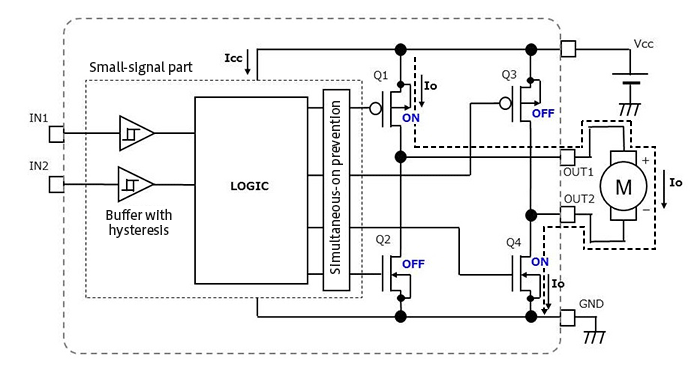Technical Information Site of Power Supply Design

• TECH INFO
• Motor Notes : Method of Calculating the Power Consumption of a Brushed Motor Driver: Part 1

Engineer Column

Motor Notes

# Method of Calculating the Power Consumption of a Brushed Motor Driver: Part 1

Keyword rushed motor driver Motor Motor driver Motor driver power consumption Calculation of motor driver power consumption Motor driver current consumption Motor driving Motor power consumption

In this column, I would like to consider the power consumption of motor drivers.

I have been asked whether the following may be used to calculate the power consumption of a motor driver.

(IC circuit current + current flowing in motor) × power supply voltage

At first glance, this might seem to be correct, but it is not. “IC circuit current × power supply voltage” is correct, but ”current flowing in motor × power supply voltage” includes the power consumption of the motor. Hence the output power consumption of the motor driver IC should be found, and then added to the power consumption of the IC circuit.

The output power consumption is calculated as "loss voltage × output current". We'll later describe calculations for the H-bridge that is the output stage of the motor driver, but as a simple example to aid understanding, we'll show the calculation equation for the power consumption of a linear regulator IC.

Power consumption of linear regulator IC = the IC's own power consumption (Vin×Iin) + input/output voltage difference (Vin-Vout) × output current (IO)

As Iin in this equation, a current such as Icc or Iq given in the data sheet is used; this is the current from the Vin pin that flows to an output voltage regulation circuit within the IC and is consumed. The term following this, "input/output voltage difference × output current", is the power loss (consumption) of the output.

For example, when the output current of the op-amp IC does not reach milliamp level, the product of the power supply voltage and the power supply current can be used without resulting in an error with any great impact. However, in the case of an IC that supplies a comparatively large output current, such as a power supply IC or a motor driver IC, if the calculation "power supply voltage × output current" is used, a substantial error results.

We have gone off on a tangent somewhat, but the same type of reasoning applies when calculating the power consumption of motor driver ICs.

## Method of Calculating the Power Consumption of a Brushed Motor Driver

Well then, taking as an example a brushed motor driver IC, we explain equations to calculate power consumption. Representative methods for driving brushed motors include constant-voltage driving and PWM driving; we begin with an explanation for the case of constant-voltage driving.

① Equations to calculate power consumption for constant-voltage driving

In essence, the power consumption Pc of a motor driver IC using constant-voltage driving can be determined from the following equation.

Pc = small-signal circuit current × power supply voltage + current flowing in motor × (voltage across high-side outputs + voltage across low-side outputs)

Below is a schematic diagram to show where power consumption occurs.The part labeled "small-signal part" is the control circuit of the motor driver IC; in this part, the circuit current is Icc, the power supply voltage is Vcc, and the current flowing in the motor is IO. The MOSFETs Q1 to Q4 form the H-bridge circuit in the output stage.

The power consumption of the small-signal circuit can be found simply from Icc×Vcc.

In the H-bridge, the high-side MOSFET Q1 and the low-side MOSFET Q4 are turned on, and IO flows from Vcc, through Q1 to the motor, and through Q4 to GND. We determine the power consumption (loss) of the H-bridge at this time. In the on-state, a MOSFET can be considered to be equivalent to a resistor, with the resistance value equal to the MOSFET on-resistance Ron. If the on-resistance of the high-side MOSFET Q1 is RonH and the on-resistance of the low-side MOSFET Q4 is RonL, then the voltages across the outputs can be represented by the following equations.

Voltage across high-side outputs VH = IO × RonH
Voltage across low-side outputs VL＝IO × RonL

From these equations, the power consumption Pc can be calculated using the following equation.

Pc＝Icc×Vcc＋Io×(VH＋VL)＝Icc×Vcc＋IO2×(RonH＋RonL)

A method in which the actual VH and VL are measured is more pragmatic, but the Ron for MOSFETs is provided on the data sheets, and so if the maximum values are used in calculations, worst-case estimates can be made.

(to be continued)